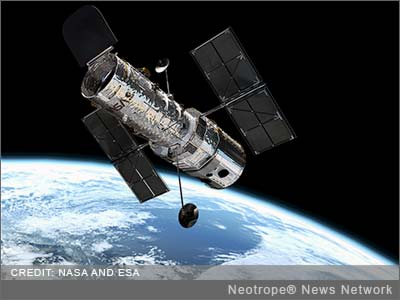SHAREMONTREAL, Quebec /eNewsChannels/ — NEWS: According to the Pantheory Research Organization, a new study of type 1a supernova data has concluded, for the first time, that dark energy probably does not exist. The paper is entitled “An Alternative Universe-Scale Analytic Metrology and Related Cosmological Model.” The paper was published in the February 2014 issue of the journal of Applied Physics Research, by the Canadian Center of Science and Education and authored by Forrest W. Noble and Timothy M. Cooper.

This new research study involves “an analysis of type 1a supernovae data with newly proposed distance and brightness equations which, if valid, would eliminate the need for changing expansion rates of the universe vis-a-vis dark energy.”

“Major conclusions of the study were that the observable universe is probably not expanding also precluding its accelerated expansion (dark energy), and that the Hubble Distance Formula is wrong and needs to be replaced,” say authors Noble and Cooper.

This conclusion was based upon researchers comparing Big Bang related Hubble formula distance calculations with alternative-cosmology derived distance and brightness equations and related calculations, concerning a present-day study of type 1a supernova observations.

The subject paper used the same type 1a supernovae data as the original studies and papers that proposed the accelerated expansion of the universe, but this and other present-day studies now include much more data since the quantity of international observations of type 1a supernovae has multiplied greatly since then. At the time of the original studies in the 1990’s that proposed the accelerated expansion of the universe, there were at least two unstated assumptions being made.

The first well-accepted assumption then and now was that space is expanding, and the second assumption was that the Hubble Formula calculates distances correctly based upon a constant expansion rate of the universe. The Hubble Distance Formula was derived from the Special Theory of Relativity based upon an expanding universe. The authors of this paper say that if either or both of these assumptions were wrong, then dark energy could be an invalid hypothesis even though the Nobel Prize was granted for its discovery.

The non-standard cosmology used as the basis for deriving the alternative equations and calculations used for comparison in the study is called the “Pan Theory.”